### Wave Optics Class 12th Physics Part Ii CBSE Solution

##### Question 1.The 6563 Å Hα line emitted by hydrogen in a star is found to be red shifted by 15 Å. Estimate the speed with which the star is receding from the Earth.Answer:Redshift is a Doppler’s effect phenomena seen in the case of electromagnetic waves, It happens whenever the light source moves away from the observer or vice versa and the light or other electromagnetic radiation from an object seems to be increased in wavelength, or shifted to the red end of the spectrum. The wavelength of the light is observed increased because of the change in the frequency of the light observed, since the departing source will give an impression of decreased frequency or increased wavelength, the light observed will shift towards red spectrum or the larger wavelength.The relation of red shift with velocity is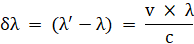Where,λ’ is the apparent increased wavelength,λ is the original wavelengthδλ is the difference between apparent and original wavelength.Given:Wavelength of Hα line emitted by hydrogenλ = 6563 Å = 6563 × 10 -10 m.Red shift of the star, δλ = (λ’ - λ) = 15 Å = 15 × 10- 10 mSpeed of Light, c = 3 × 108 m/sWe suppose the velocity of the star receding away from the Earth as v.Since, the ratio of apparent change in the wavelength is equal to the ratio of velocity of receding body and the standard speed of light.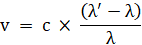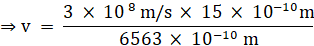⇒ v = 6.87 × 105 m/sThe estimatedspeed with which the star is receding from the Earth is 6.87 × 105 m/sQuestion 2.Explain how Corpuscular theory predicts the speed of light in a medium, say, water, to be greater than the speed of light in vacuum. Is the prediction confirmed by experimental determination of the speed of light in water? If not, which alternative picture of light is consistent with experiment?Answer:Newton’s Corpuscular theory of light theorised the light to consist of small corpuscles and states that, when light corpuscles strike the interface of two media from a rarer (here air) to denser (water) medium, the particles experience forces of attraction normal to the surface. Hence, component of the velocity normal to the point of interaction with the surface increases while the component parallel along the surface remains unchanged. Thus component of velocity of light in one direction will be equal.Suppose we takev as the velocity of the light in rarer medium (air)v’ as velocity in the denser medium (water)The components parallel to the surfaces can be written as,v sini and v’sinrwhere,i is the angle of incidence in the rarer medium,r is the angle of refraction in the denser mediumBut, v sin(i) = v’ sin(r)⇒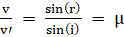(where μ is the refractive index). And, we have refractive index ‘μ’ always greater than unity.Thus, light should travel faster in the denser medium like water than in air.Note: The experimental results prove that no object can travel faster than the speed of light i.e. c.Thus,No, the predictions of Newton’s Corpuscular theory were not confirmed by any experimental determination of the speed of light in water.The Huygens wave theory predicts that v’ is greater than v which is consistent with the experimental results.Question 3.You have learnt in the text how Huygens’ Principle leads to the laws of reflection and refraction. Use the same principle to deduce directly that a point object placed in front of a plane mirror produces a virtual image whose distance from the mirror is equal to the object distance from the mirror.Answer:Huygens principle is used to explain phenomena such as refraction, reflection and diffraction by describing the light to travel forward in the form of wave fronts. These primary wave fronts act as source for the next secondary one in the propagation of light.We start by supposing that an object O placed in front of a plane mirror MP at a distance equal to the radius of the circle (as shown in the figure below)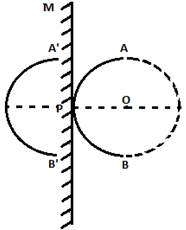A circle is drawn from the centre taken as object O such that the plane mirror is just tangent to the circle at the point P. The Huygens’s Principle thus points that AB is the first wave front of the incident light from the object O.If we suppose the absence of the mirror, then by Huygens principle a similar wave front can be drawn parallel to AB behind the P at a distance of the same radius of the circle (as given in the figure below).A’B’ can be considered as a virtual reflected ray for the plane mirror. Therefore, a point object placed in front of the plane mirror produces a virtual image whose distance from the mirror is equal to the object at an equal distance. Further another secondary wave front can be drawn by taking the circle A’B’ and placing a mirror tangent to the circle and parallel to the previous one. The point ‘P’ will act as an object for the next virtual image. The secondary wave fronts thus are at a distance of the radius of the circle from the tangent of a virtual plane mirror.Question 4.Let us list some of the factors, which could possibly influence the speed of wave propagation:(i) Nature of the source.(ii) Direction of propagation.(iii) Motion of the source and/or observer.(iv) Wavelength.(v) Intensity of the wave.On which of these factors, if any, does(a) The speed of light in vacuum,(b) The speed of light in a medium (say, glass or water), depend?Answer:(a) The speed of light in vacuum i.e. 3 × 108 m/s is universal constant and thus, it’s value stays the same in vacuum as there are no interacting particles, It is not effected by (i) the nature of the source, (ii) Direction of propagation (iii) Motion of the source or observer (iv) Wavelength and (v) Intensity of the wave.Thus, the given factors will have no effect on the speed of light in vacuum.(b) Since, the factors such as (i) the nature of the source (ii) the direction of propagation (iv) wavelength change the intrinsic property of the light but other factors given are external factors and does not affect the propagation property of light. Thus, The speed of light in a medium like glass or water is influenced only by (i) (ii), (iv).Question 5.For sound waves, the Doppler formula for frequency shift differs slightly between the two situations: (i) source at rest; observer moving, and (ii) source moving; observer at rest. The exact Doppler formulas for the case of light waves in vacuum are, however, strictly identical for these situations. Explain why this should be so. Would you expect the formulas to be strictly identical for the two situations in case of light travelling in a medium?Answer:(i) No, the properties of propagation of light and sound are very different from each other. Sound waves can propagate only in the presence of a medium. The velocities do not just simply add up when they approach the speed of light.(ii) The two situations whether it is source at rest or observer at rest are not identical because the sound waves are mechanical and thus depends on the properties of the medium through which it is travelling.In case of the observer moving the apparent change in the frequency can be given as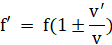In case of source moving the apparent change frequency can be given as,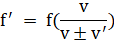Where,f is the frequency of waves from sourcef’ is the apparent frequency observed.ν’ is the speed of the observer and ν is the speed of the wave from the sourceThe negative sign is for the observer or source moving away from the one at rest. The positive direction is for when the distance between them is decreasing.Hence, The Doppler’s formulas for the two situations cannot be the same.(iii) In case of the light waves, In vacuum, the above situations (a) and (b) are identical because the speed of light is a universal constant and does on depend on the motion of the observer or the source and thus the value always remains the same. But when light travels in a medium, according to the wave theory of light the speed depends on the nature of the medium.Question 6.In double - slit experiment using light of wavelength 600 nm, the angular width of a fringe formed on a distant screen is 0.1°. What is the spacing between the two slits?Answer:Angular width is related to the slit spacing (d) as:θ = λ/dThus,d = λ/θWhere,λ is the wavelength of lightθ is the angular width of the fringe on the screend is the spacing between the two slits.Given,Wavelength of light, λ = 600 nm = 600 × 10 - 9 mThe angular width of the fringe θ = 0.1°Note: The given angular width is in degrees, We must convert degrees into radiant for the angular width of the fringe.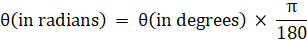Thus, Angular width, θ of the fringe can be written in radians as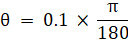⇒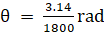d =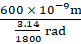⇒ d = 3.44 × 10 - 4 mThe spacing between the two slits “d” is 3.44 × 10 - 4 mQuestion 7.Answer the following question:In a single slit diffraction experiment, the width of the slit is made double the original width. How does this affect the size and intensity of the central diffraction band?Answer:If the width of the slit is made double the original width in a single slit diffraction experiment, the size of the central diffraction band will become half of the original value.Since, we have the first minima occurs atb sinθ = nλIf we take n = 1, for the central maxima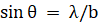Where,θ is the angle between the wave ray at the point on the screen and the normal at the centre of the slit.The space between the first minimums contains the central maxima.If we increase the width will thus decrease.The intensity depends on the amplitude asI ∝ A2Thus, when we double the size of the slit we double the amplitude and hence, the intensity becomes four times that of original intensity.When central maxima are reduced to half, logically the same light has to be distributed in a smaller region. Increasing the intensity of central band maxima will make the intensity four times that of the original intensity.Question 8.Answer the following question:In what way is diffraction from each slit related to the interference pattern in a double - slit experiment?Answer:If we close on of the slits in the case of a double slit experiment, the given apparatus now work for a single slit diffraction experiment. The central maxima and other secondary maxima from each individual single slits interfere to form a central maxima and other maxima for the double slit experiment due to their path differences when these slits work together as a coherent source.Question 9.Answer the following question:When a tiny circular obstacle is placed in the path of light from a distant source, a bright spot is seen at the centre of the shadow of the obstacle. Explain why?Answer:The diffraction pattern is observed when the size of the object is comparable to the wavelength of the diffracting light.When a tiny circular obstacle is placed in the path of light from a distant source, a bright spot is seen at the centre of the shadow of the obstacle. This bright spot is a central maxima observed in the case of diffraction from the single slit. This is because light waves are diffracted from the edge of the tiny circular obstacle, which interferes constructively at the centre of the shadow. This constructive interference produces a bright spot.Question 10.Answer the following question:Two students are separated by a 7 m partition wall in a room 10 m high. If both light and sound waves can bend around obstacles, how is it that the students are unable to see each other even though they can converse easily?Answer:Bending the waves by obstacles at a large considerable angle only if the size of the obstacle is comparable to the wavelength of the waves.If the wavelength of light waves is too small compared to the size of the obstacle, then the diffraction would not be considerable. Hence, the students are unable to see each other. On the other hand, the size of the wall is comparable to the wavelength of the sound waves. Thus, the bending of the waves takes place at a large angle. Hence, the students are able to hear each other.Question 11.Answer the following question:Ray optics is based on the assumption that light travels in a straight line. Diffraction effects (observed when light propagates through small apertures/slits or around small obstacles) disprove this assumption. Yet the ray optics assumption is so commonly used in understanding location and several other properties of images in optical instruments. What is the justification?Answer:The ray optics assumption is used in understanding location and several other properties of images in optical instruments because in the commonly used optical instruments are large when compared to the wavelength of light. Thus, the diffraction, a phenomenon is not observed when light waves interact on such a macro level and there is no violation of rectilinear property of light.Question 12.Two towers on top of two hills are 40 km apart. The line joining them passes 50 m above a hill halfway between the towers. What is the longest wavelength of radio waves, which can be sent between the towers without appreciable diffraction effects?Answer:Fresnel’s distance is given by the relation, Zp = a2 / λWhere,λ is the wavelength of the radio wavesZp is the Fresnel distance, which shows that on distance smaller than this, the spreading due to diffraction smaller compared to the size of the beam.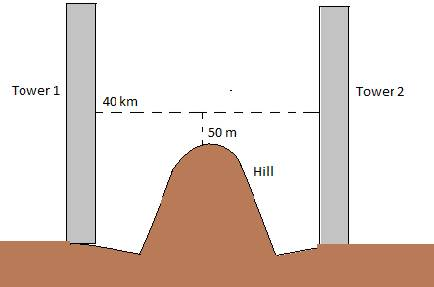If we want to prevent diffraction of the radio waves , According to the validity of ray optics, the distance of the middle should be less than The size of aperture “a” = 50 mZ= 20 km = d/2 = 2 × 104 mAperture can be taken as:a = 50 mThe longest wavelength of radio waves which can be sent without any hindrance due to diffraction effect,λ = a2 / Zp⇒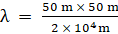⇒ λ = 1250 × 10 - 4 m = 12.5 cmTherefore, the longest wavelength of the radio waves is 12.5 cmQuestion 13.A parallel beam of light of wavelength 500 nm falls on a narrow slit and the resulting diffraction pattern is observed on a screen 1 m away. It is observed that the first minimum is at a distance of 2.5 mm from the centre of the screen. Find the width of the slit.Answer:The relation between fringe width “x” and the order of minima “n” is given as: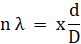or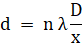where,d = width of the screenD = distance between the screen and the slitn = order of minimaλ = wavelength of the lightGiven,λ = 500 nm = 500 × 10 - 9 mDistance between the screen and the slit , D = 1 mFor first minima, n = 1x = 2.5 mm = 2.5 × 10 - 3 mDistance between the slits = dDistance of the first minimum from the centre of the screen can be obtained as: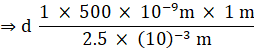⇒ d = 2 × 10 - 4 m = 0.2 mmTherefore, the width of the slits is 0.2 mm.Question 14.Answer the following question:When a low flying aircraft passes overhead; we sometimes notice a slight shaking of the picture on our TV screen. Suggest a possible explanation.Answer:Flying aircrafts transmits radar waves. When a low flying aircraft passes overhead, this radar signal however weak, can be received by the antenna and thus interferes with the TV signals. Thus, this interference causes a slight shaking of the picture that we sometimes notice on our TV screen.Question 15.Answer the following question:As you have learnt in the text, the principle of linear superposition of wave displacement is basic to understanding intensity distributions in diffraction and interference patterns.What is the justification of this principle?Answer:The linear superposition describes the linear character and solutions of the differential equation that governs the wave motion. The intensity distributions in both diffraction and interference patterns require the linear superposition to deduce and characterize the wave equation. If we take X1 and X2 as the displacement covered by the two waves. We can infer the resultant displacement of the two waves given as, X = X1 + X2Question 16.In deriving the single slit diffraction pattern, it was stated that the intensity is zero at angles of nλ/a. Justify this by suitably dividing the slit to bring out the cancellation.Answer:The path difference between the beams at each ends of the slits can be written asbsinθ = nλwhere,b is the size of the slit,n is the order of the minimaθ is the phase angle.The observations state that the intensity is zero at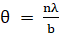First, Let us divide the slit into two equal parts. Then a path a difference of λ/2 i.e. π is introduced between the waves of each part. The waves then superimpose to interfere destructively and thus producing a zero intensity minima on the screen. We can thus observe the same results if we divide the screen accordingly for n = 2,3,4….

PDF FILE TO YOUR EMAIL IMMEDIATELY PURCHASE NOTES & PAPER SOLUTION. @ Rs. 50/- each (GST extra)

HINDI ENTIRE PAPER SOLUTION

MARATHI PAPER SOLUTION

SSC MATHS I PAPER SOLUTION

SSC MATHS II PAPER SOLUTION

SSC SCIENCE I PAPER SOLUTION

SSC SCIENCE II PAPER SOLUTION

SSC ENGLISH PAPER SOLUTION

SSC & HSC ENGLISH WRITING SKILL

HSC ACCOUNTS NOTES

HSC OCM NOTES

HSC ECONOMICS NOTES

HSC SECRETARIAL PRACTICE NOTES

# 2019 Board Paper Solution

HSC ENGLISH SET A 2019 21st February, 2019

HSC ENGLISH SET B 2019 21st February, 2019

HSC ENGLISH SET C 2019 21st February, 2019

HSC ENGLISH SET D 2019 21st February, 2019

SECRETARIAL PRACTICE (S.P) 2019 25th February, 2019

HSC XII PHYSICS 2019 25th February, 2019

CHEMISTRY XII HSC SOLUTION 27th, February, 2019

OCM PAPER SOLUTION 2019 27th, February, 2019

HSC MATHS PAPER SOLUTION COMMERCE, 2nd March, 2019

HSC MATHS PAPER SOLUTION SCIENCE 2nd, March, 2019

SSC ENGLISH STD 10 5TH MARCH, 2019.

HSC XII ACCOUNTS 2019 6th March, 2019

HSC XII BIOLOGY 2019 6TH March, 2019

HSC XII ECONOMICS 9Th March 2019

SSC Maths I March 2019 Solution 10th Standard11th, March, 2019

SSC MATHS II MARCH 2019 SOLUTION 10TH STD.13th March, 2019

SSC SCIENCE I MARCH 2019 SOLUTION 10TH STD. 15th March, 2019.

SSC SCIENCE II MARCH 2019 SOLUTION 10TH STD. 18th March, 2019.

SSC SOCIAL SCIENCE I MARCH 2019 SOLUTION20th March, 2019

SSC SOCIAL SCIENCE II MARCH 2019 SOLUTION, 22nd March, 2019

XII CBSE - BOARD - MARCH - 2019 ENGLISH - QP + SOLUTIONS, 2nd March, 2019

# HSCMaharashtraBoardPapers2020

(Std 12th English Medium)

HSC ECONOMICS MARCH 2020

HSC OCM MARCH 2020

HSC ACCOUNTS MARCH 2020

HSC S.P. MARCH 2020

HSC ENGLISH MARCH 2020

HSC HINDI MARCH 2020

HSC MARATHI MARCH 2020

HSC MATHS MARCH 2020

# SSCMaharashtraBoardPapers2020

(Std 10th English Medium)

English MARCH 2020

HindI MARCH 2020

Hindi (Composite) MARCH 2020

Marathi MARCH 2020

Mathematics (Paper 1) MARCH 2020

Mathematics (Paper 2) MARCH 2020

Sanskrit MARCH 2020

Sanskrit (Composite) MARCH 2020

Science (Paper 1) MARCH 2020

Science (Paper 2)

Geography Model Set 1 2020-2021

MUST REMEMBER THINGS on the day of Exam

Are you prepared? for English Grammar in Board Exam.

Paper Presentation In Board Exam

How to Score Good Marks in SSC Board Exams

Tips To Score More Than 90% Marks In 12th Board Exam

How to write English exams?

How to prepare for board exam when less time is left

How to memorise what you learn for board exam

No. 1 Simple Hack, you can try out, in preparing for Board Exam

How to Study for CBSE Class 10 Board Exams Subject Wise Tips?

JEE Main 2020 Registration Process – Exam Pattern & Important Dates

NEET UG 2020 Registration Process Exam Pattern & Important Dates

How can One Prepare for two Competitive Exams at the same time?

8 Proven Tips to Handle Anxiety before Exams!